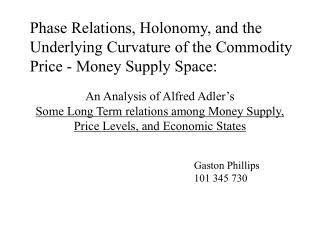DownloadDownload PresentationGaston Phillips 101 345 730

Gaston Phillips 101 345 730

Télécharger la présentationGaston Phillips 101 345 730

- - - - - - - - - - - - - - - - - - - - - - - - - - - E N D - - - - - - - - - - - - - - - - - - - - - - - - - - -
Presentation Transcript

1. Phase Relations, Holonomy, and the Underlying Curvature of the Commodity Price - Money Supply Space: An Analysis of Alfred Adler’sSome Long Term relations among Money Supply, Price Levels, and Economic States Gaston Phillips 101 345 730

2. US Money Supply (In Billions)

3. Annual Average Commodity Price Index

4. M1 vs WPI

5. Holonomy 1 • Consider a Triangle whose sides are on great circles of a sphere. • The three vertices are all right angles

6. Holonomy 2 • Draw a vector (here in yellow), on the surface of the sphere.

7. Holonomy 3 • As the vector moves to the second vertex, it remains parallel to the first side and perpendicular to the second.

8. Holonomy 4 • As the vector moves to the third vertex, it remains perpendicular to the second side, so it’s parallel to the third.

9. Holonomy 5 • Now as it moves back to the first vertex it remains parallel to the third side, so it ends up perpendicular to the first, rotated 90 degrees from its original orientation.

10. Log-Scaled Commodity Price

11. Periodicity Modulo 55

12. Phase Rule dG/dt > 0 and dm/dt > dM/dt then dc/dt = dG/dt dG/dt > 0 and dm/dt = dM/dt then dc/dt = dG/dt dG/dt > 0 and dm/dt < dM/dt then * dG/dt = 0 and dm/dt > dM/dt then dc/dt = dG/dt dG/dt = 0 and dm/dt = dM/dt then dc/dt = dG/dt dG/dt = 0 and dm/dt < dM/dt then * dG/dt < 0 and dm/dt > dM/dt then dc/dt = F(t) dm/dt dG/dt < 0 and dm/dt = dM/dt then dc/dt = dG/dt dG/dt < 0 and dm/dt < dM/dt then dc/dt = dG/dt

13. Log-Scaled Money Supply

14. Change in log-scaled Money

15. Test Statistic

16. Error by Phase

17. To Do • Hypothesis Test • Determine Power of Experiment • Explain Holonomy Better • MSE, S2, &c